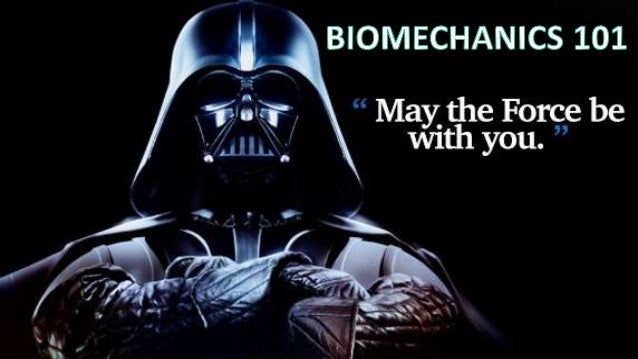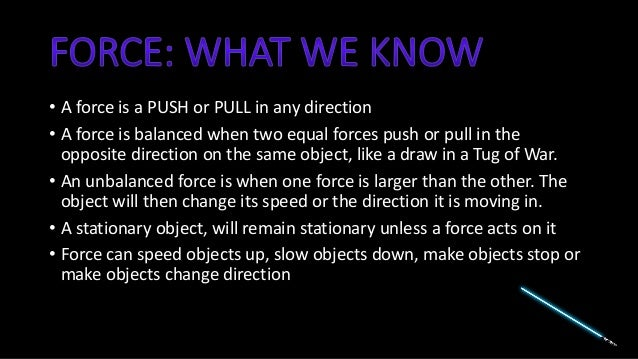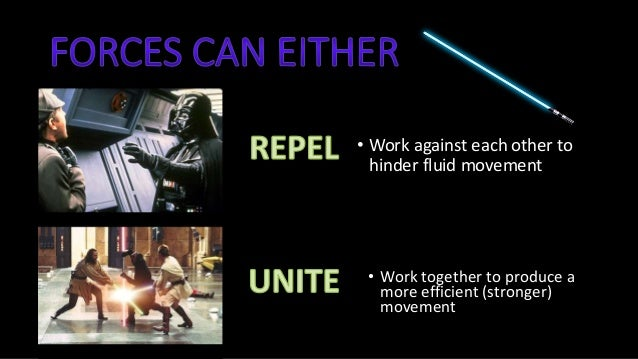Successfully reported this slideshow.Upcoming SlideShare
×

ofUpcoming SlideShare
Prelim PDHPE Core 2: Body in Motion
Next

2 Likes

Share

# Biomechanics 101: Motion & Force

Outcomes covered:
P9 describes biomechanical factors that influence the efficiency of the body in motion

See all

See all

### Biomechanics 101: Motion & Force

1. 1. • A force is a PUSH or PULL in any direction • A force is balanced when two equal forces push or pull in the opposite direction on the same object, like a draw in a Tug of War. • An unbalanced force is when one force is larger than the other. The object will then change its speed or the direction it is moving in. • A stationary object, will remain stationary unless a force acts on it • Force can speed objects up, slow objects down, make objects stop or make objects change direction
2. 2. • Work against each other to hinder fluid movement • Work together to produce a more efficient (stronger) movement
3. 3. • In PDHPE these ‘forces’ have specific names that either work to enhance movement or can hinder movement • They can impact both the athlete and the equipment they use • For example, in soccer when you kick a ball. Most obvious is that the athlete applies a force on the ball. However, many forces are in play… • Internal forces: muscles causing movement, mass of ball • External forces: gravity, wind resistance, rotational force
4. 4. • In PDHPE you only need to recall the ones in the syllabus!! • If you have a sport specific question (eg. Discus), you only need to discuss the forces that impact that specific sport!!!
5. 5. • Motion describes movement and path of a body • Linear motion, velocity, speed, acceleration, momentum • NO! • Only, linear motion, acceleration & momentum
6. 6. PEE CHAIN – (POINT)DEFINE, EXAMPLE, EXPLAIN • Linear motion occurs when a body and all parts connected to it travel the same distance in the same direction and at the same speed. • Acceleration to increase speed quickly • Momentum quantity of force a body contains. (M=mv) • Aspects that impact momentum - mass of body, location of mass, angular v linear momentum
7. 7. • Balance and stability impacts both the athlete and sometimes the equipment used (ie. Discus)
8. 8. PEE CHAIN – (POINT)DEFINE, EXAMPLE, EXPLAIN • A solid base of support for discus thrower is integral because…
9. 9. • The body can apply and absorb force For every force there is an equal and opposite force • This means for every action/movement there are two forces in play. • For every force there is a reaction force that is equal in size, but opposite in direction • A force can be transferred from one body to another
10. 10. • Power (biomechanics) is the ability of muscle groups to contract at speed. • An increase in strength (force) or an increase in the speed at which muscles shorten results in an increase in power. • Jumpers and runners need to focus on rapid muscular contraction while controlling the strength aspect. This is called speed-dominated power. In contrast, the weight-lifter needs power and must be able to lift the weight. He or she needs to develop strength-dominated power.
11. 11. There are principles to remember with the application of force on an object: 1)The quantity of force applied to the object is important. The greater the force, the greater is the acceleration of the object 2)If the mass of an object is increased, more force is needed to move the object the same distance. For example, if a football becomes heavier as a result of wet conditions, more force is required to pass or kick it. 1)Objects of greater mass require more force to move them than objects of smaller mass. The size of the discus, javelin and shot-put is smaller for younger students than older students. This assumes that older students have greater mass and are thereby able to deliver more force than younger students because of their increased size (mass) and (possibly) strength.
12. 12. In many sports and activities, the body rotates about an axis. When this happens centripetal force and centrifugal force are experienced. Centripetal force is a force directed towards the centre of a rotating body. Centrifugal force is a force directed away from the centre of a rotating body. These forces commonly occur with skills that require rotation such as the golf swing or the hammer throw.
•#### EmilyElizabeth10

Mar. 13, 2019
•#### SarahGraham11

Jul. 18, 2016

Total views

9,934

On Slideshare

0

From embeds

0

Number of embeds

5,107

61

Shares

0